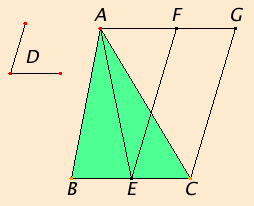# Proposition 42

To construct a parallelogram equal to a given triangle in a given rectilinear angle.

Let ABC be the given triangle, and D the given rectilinear angle.

It is required to construct in D a parallelogram equal to the triangle ABC in the rectilinear angle.Bisect BC at E, and join AE. Construct the angle CEF on the straight line EC at the point E on it equal to the angle D. Draw AG through A parallel to EC, and draw CG through C parallel to EF.

Then FECG is a parallelogram.

I.38

Since BE equals EC, therefore the triangle ABE also equals the triangle AEC, for they are on equal bases BE and EC and in the same parallels BC and AG. Therefore the triangle ABC is double the triangle AEC.

But the parallelogram FECG is also double the triangle AEC, for it has the same base with it and is in the same parallels with it, therefore the parallelogram FECG equals the triangle ABC.

And it has the angle CEF equal to the given angle D.

Therefore the parallelogram FECG has been constructed equal to the given triangle ABC, in the angle CEF which equals D.

Q.E.F.

## Guide

The idea of the construction is as follows. First make a triangle half the size of the given triangle. Next skew the half-size triangle to make one of its angles the desired angle without changing its area. Complete the resulting half-size triangle to a parallelogram. that’s the desired parallelogram equal to the original triangle in the desired angle.

#### Application of areas

With this proposition Euclid moves to the next phase in his study of areas, the application of areas. Before this, he has exhibited various situations when triangles or parallelograms have equal areas, or when a triangle has half the area of a parallelogram. But now he’s interested in constructing another figure with the same area as a given figure.

In this proposition, he constructs a parallelogram that has a given angle and has the same area as a given triangle. But his goals are coming up, application of areas in I.45 and quadrature in II.14. In proposition I.45, given a rectilinear figure an equal parallelogram is constructed on a given side within a given angle. This kind of construction is called “applying” an area to a side. The area is sort of laid along the line. It may be that before Euclid the area was always applied to a rectangle along the line, but Euclid generalized the construction to parallelograms. This extra generalization is not often used.

Later, in proposition II.14 a square is constructed equal to a given rectilinear figure, a process called quadrature (making into a square) of the figure. This square is a canonical measure of the area.

#### Use of Proposition 42

This construction is used as part of the constructions in the two propositions following the next one.